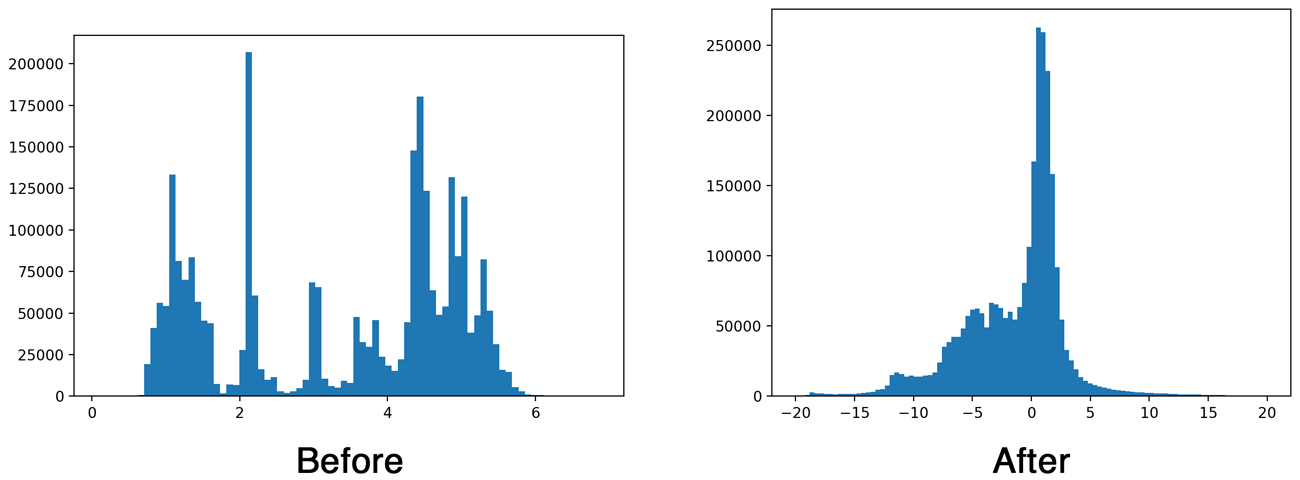# Conv层BN层合并

## BN层合并

converting-to-metal

However, there was a small wrinkle… YOLO uses a regularization technique called batch normalization after its convolutional layers.

The idea behind “batch norm” is that neural network layers work best when the data is clean. Ideally, the input to a layer has an average value of 0 and not too much variance. This should sound familiar to anyone who’s done any machine learning because we often use a technique called “feature scaling” or “whitening” on our input data to achieve this.

Batch normalization does a similar kind of feature scaling for the data in between layers. This technique really helps neural networks perform better because it stops the data from deteriorating as it flows through the network.

To give you some idea of the effect of batch norm, here is a histogram of the output of the first convolution layer without and with batch normalization:Batch normalization is important when training a deep network, but it turns out we can get rid of it at inference time. Which is a good thing because not having to do the batch norm calculations will make our app faster. And in any case, Metal does not have an MPSCNNBatchNormalization layer.

Batch normalization usually happens after the convolutional layer but before the activation function gets applied (a so-called “leaky” ReLU in the case of YOLO). Since both convolution and batch norm perform a linear transformation of the data, we can combine the batch normalization layer’s parameters with the weights for the convolution. This is called “folding” the batch norm layer into the convolution layer.

Long story short, with a bit of math we can get rid of the batch normalization layers but it does mean we have to change the weights of the preceding convolution layer.

A quick recap of what a convolution layer calculates: if x is the pixels in the input image and w is the weights for the layer, then the convolution basically computes the following for each output pixel:

out[j] = x[i]*w + x[i+1]*w + x[i+2]*w + ... + x[i+k]*w[k] + b


This is a dot product of the input pixels with the weights of the convolution kernel, plus a bias value b.

And here’s the calculation performed by the batch normalization to the output of that convolution:

        gamma * (out[j] - mean)
bn[j] = ---------------------- + beta
sqrt(variance)


It subtracts the mean from the output pixel, divides by the variance, multiplies by a scaling factor gamma, and adds the offset beta. These four parameters — mean, variance, gamma, and beta — are what the batch normalization layer learns as the network is trained.

To get rid of the batch normalization, we can shuffle these two equations around a bit to compute new weights and bias terms for the convolution layer:

           gamma * w
w_new = --------------
sqrt(variance)

gamma*(b - mean)
b_new = ---------------- + beta
sqrt(variance)


Performing a convolution with these new weights and bias terms on input x will give the same result as the original convolution plus batch normalization.

Now we can remove this batch normalization layer and just use the convolutional layer, but with these adjusted weights and bias terms w_new and b_new. We repeat this procedure for all the convolutional layers in the network.

Note: The convolution layers in YOLO don’t actually use bias, so b is zero in the above equation. But note that after folding the batch norm parameters, the convolution layers do get a bias term.

Once we’ve folded all the batch norm layers into their preceding convolution layers, we can convert the weights to Metal. This is a simple matter of transposing the arrays (Keras stores them in a different order than Metal) and writing them out to binary files of 32-bit floating point numbers.

If you’re curious, check out the conversion script yolo2metal.py for more details. To test that the folding works the script creates a new model without batch norm but with the adjusted weights, and compares it to the predictions of the original model.

08-01
03-092646
01-29734
03-181万+
09-278578
01-042万+
09-29449
12-024440
05-201204
11-031206
03-13413
01-034371
04-02382
01-246995
08-065394
09-183384
05-203720

### “相关推荐”对你有帮助么？

•非常没帮助
•没帮助
•一般
•有帮助
•非常有帮助被折叠的  条评论 为什么被折叠?到【灌水乐园】发言点击重新获取扫码支付1.余额是钱包充值的虚拟货币，按照1:1的比例进行支付金额的抵扣。
2.余额无法直接购买下载，可以购买VIP、C币套餐、付费专栏及课程。余额充值